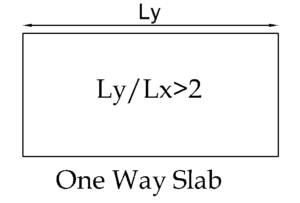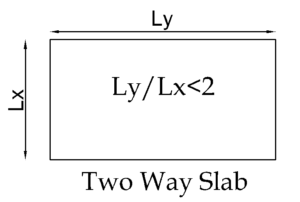# How to Calculate Steel Quantity for Slab? – Reinforcement Calculation

In this post, we are going to explain how to calculate steel quantity for the slab? For both one way and two way slab with an example.

Note: For Better View, Please read this post in landscape mode if you are on the mobile device.

We hope you have already familiar with

Quick Recap,

 One Way Slab Ly/Lx > 2Two Way Slab Ly/Lx < 2# Slab Reinforcement Calculation

## One way slab Reinforcement Detailing

The Biggest misconception is considering beam outer to outer as slab span. Please refer the below diagramLet’s take an example of the below one way slab diagramGiven

• Main bars are 12 mm in diameter @ 150 mm centre to centre spacing
• Distribution bars are 8 mm in diameter @ 150 mm centre to centre spacing. (Main Bar & Distribution Bar Difference)
• Top and Bottom Clear Cover is 25 mm
• Consider Development length as 40 d
• Thickness of Slab – 150 mm

### One Way Slab Bar Bending Schedule Calculation

Step 1

FIrst, find number of rods required for main reinforcement and distribution

Number of Bars Formula = (Length of slab / spacing) + 1

Number of Main Bars = (Ly / spacing) + 1 = (5000/150) + 1 = 34 nos

Number of Distribution Bars = (Lx / spacing) + 1 = (2000 / 150) + 1 = 14 nos

Step 2

Find cutting length of main bars and distribution barsCutting Length of Main Bar,

= Clear Span of Slab (Lx) + (2 X Developement Length) +(1 x Inclined length) – (45° bend x 2)

Crank Length = 0.42 D, We have already discussed this on Cutting Length of Main Bar post

= 2000 + (2 x 40 x 12) + (1 x 0.42 x D) – (1d x 2)

= 2000 + 960 + 0.42D – (1x12x2) = 2960 +0.42D – 24

D = Slab thickness – 2 side clear cover – dia of bar =  150 – 50 -12 = 88 mm

Length of Main Bar = 2960+(0.42 x 88) – 24 = 2973 mm or 2.97 m

Step 3

Find length of distribution bar

= Clear Span (Ly) + (2 x Development Length (Ld))

= 5000 + (2 x 40 x 8) = 5640 mm or 5.64 m

Step 4

Find Top Bar (Extra) ; Top Bars are provided at the top of critical length (L/4) area, Please refer the drawing section A-A

Number of top bars = (Lx/4) / spacing + 1  = (2000/4) / 150 +1 = 4 Nos x 2 sides = 8 Nos

Length of Top Bar (L) = same as distribution bars = 5.64 m

### Bar Bending Schedule for One Way Slab

Please refer the Bar Bending Shape Code & Steel Weight Calculation post

## Two way slab Reinforcement Detailing

Now Calculate Bar Bending Schedule for Two Way Slab.

Let’s take an example the below two-way slab diagram• Main bars are 12 mm in diameter @ 150 mm centre to centre spacing
• Distribution bars are 8 mm in diameter @ 150 mm centre to centre spacing.
• Top and Bottom Clear Cover is 25 mm
• Development length – 40 d
• Thickness of Slab – 150 mm

### One Way Slab Bar Bending Schedule Calculation

Step 1

FIrst, find number of rods required for main reinforcement and distribution

Number of Required Bars Formula = (Length of slab / spacing) + 1

Number of Main Bars = Ly / spacing + 1 = (4000/150) + 1 = 27 nos

Number of Distribution Bars = Lx / spacing + 1 = 3000/150 + 1 = 21 nos

Step 2

Find cutting length of main bars and distribution barsCutting Length of Main Bar,

= Clear Span of Slab (Ly) + (1 X Developement Length) +(1 x inclined length) – (45° bend x 2)

Inclined Length = 0.42 D

= 3000 + (1 x 40 x 12) + (1 x 0.42 x D) – (1d x 2)

= 3000 + 480 + 0.42D – (1x12x2) = 3480 + 0.42 D -24

D = Slab thickness – 2 side clear cover – dia of bar =  150 – 50 -12 = 88 mm

Length of Main Bar = 3480+(0.42 x 88)-24 = 3492.9 mm or 3.49 m

Step 3

Find cutting length of distribution bar

= Clear Span of Slab (Ly) + (1 X Developement Length) +(1 x inclined length) – (45° bend x 2)

Inclined Length = 0.42 D

= 4000 + (1 x 40 x 8) + (1 x 0.42 x D) – (1d x 2)

= 4000 + 320 + (0.42×88) – (1x8x2) = 4340.96mm or 4.34 m

Step 4

Find Top Bar (Extra) ; Top Bars are provided at the top of critical length (L/4) area, Please refer the drawing section A-A

Number of Top bars on Ly side = (Lx/5) / spacing + 1  = (3000/5) / 150 +1 = 5 Nos

Length of top bar on Ly side = Clear Span of Slab (Ly) + (2 X Development Length)

= 4000+(2*40*12) = 4000+960 = 4960 mm or 4.96 m

Number of Top bars on Lx side = (Ly/5) / spacing + 1 = (4000/5) / 150 + 1 = 6 Nos

Length of top bar on Ly side = Clear Span of Slab (Ly) + (2 X Development Length)

= 3000+(2*40*12) = 3000+960 = 3960 mm or 3.96 m

### Bar Bending Schedule for Two Way Slab

NOTE

• Here we have taken development length as 40d. However, In practice, the structural drawing itself has this dimension or else you have to extend the rod up to the beam end minus clear cover.